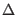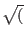Next: Josephson Tunnel Junction Model Up: Josephson Junction Model Previous: Josephson Junction Model (RSJ   Contents   Index

### RSJ Model Temperature Dependence

When the junction is not operating at the nominal temperature as specified with the tnom parameter, a correction factor is applied to the critical current to account for the temperature difference. The superconducting energy gap and therefor the junction gap voltage will also change as a function of temperature.

If the gap voltage is not specified with the vg parameter or its alias vgap, the model will compute the gap voltage from BCS theory using the superconducting transition temperature (tc parameter) and Debye temperature (tdebye parameter) at the operating temperature.

When a gap voltage is supplied, it is taken as the value at the nominal temperature. The model utilizes an interpolation formula to represent temperature variation of the gap parameter of the superconductor in the case.

In either case, the RSJ model currently assumes that the same material appears on either side of the barrier.

Below is the approximation formula for the superconducting energy gap that is employed when the nominal junction gap voltage is given explicitly. The junction vap voltage is twice the superconductor energy gap.=tanh(tcfcttc/T - 1))
Reference: https://physics.stackexchange.com/questions/192416/
interpolation-formula-for-bcs-superconducting-gap

Here,is the gap parameter, with the subscript indicating the value at temperature T=0. The tcfct parameter is semi-empirical, commonly cited as 1.74, which is the default value.

In order to apply temperature correction one takes the assumption that parameters for the reference junction are measured at nominal temperature tnom, and we therefor have=tanh(tcfcttc/tnom - 1))

The correction factor, which will multiply device gap voltage, is the ratio/. This will apply when the nominal gap voltage is given. When not given, the gap voltage is computed directly with BCS theory and the approximation formula is not used.

The critical current temperature variation again follows BCS theory. The temperature correction factor tcf multiplies the instance critical current to account for the temperature difference between operating and nominal temperatures.

tcf = (Vg/Vgnom)(tanh(eVg/4KT)/tanh(eVgnom/4KTnom))

Here, Vg is the computed gap voltage, e is the electron charge, K is Boltzmann's constant, T is temperature in Kelvin,Next: Josephson Tunnel Junction Model Up: Josephson Junction Model Previous: Josephson Junction Model (RSJ   Contents   Index
Stephen R. Whiteley 2022-09-18